# How to Use the autoimage package

## Introduction

The autoimage package makes it easy to plot a sequence of images with corresponding color scales, i.e., a sequence of heatmaps, with straightforward, native options for projection of geographical coordinates. The package makes it simple to add lines, points, and other features to the images, even when the coordinates are projected. The package allows for seamless creation of heat maps for data on regular or irregular grids, as well as data that is not on a grid.

## Examples

The most important functions in autoimage are the pimage and autoimage functions. We illustrate the basic usage of these functions using two data sets: the first is data on an irregular grid and the second is non-gridded data.

The first data set we utilize comes from the North American Regional Climate Change Assessment Program (NARCCAP, http://www.narccap.ucar.edu/). Specifically, the data are the maximum daily surface air temperature (K) (abbreviated tasmax) for the five consecutive days of May 15, 2041 to May 19, 2041 simulated using the Canadian Regional Climate Model (Caya and Laprise, 1999) forced by the Community Climate System Model atmosphere-ocean general circular model (Collins et al., 2006). The data set contains lon, a 140 $$\times$$ 115 matrix of longitude coordinates, lat, a 140 $$\times$$ 115 matrix of latitude coordinates, and tasmax, a 140 $$\times$$ 115 $$\times$$ 5 array, where each element of the third dimension of the array corresponds to the tasmax measurements of the respective day.

The second data set we utilize is geochemical measurements obtained by the United States Geological Survey (USGS) in the state of Colorado. The data are stored as a data frame with 960 rows and 31 columns. easting, northing, latitude, and longitude variables are provided in the data frame, as well as Aluminum (Al), Calcium (Ca), Iron (Fe), and many more chemical measurements.

The autoimage function is a generalization of the pimage function, so we discuss the pimage function first.

### Basic usage of pimage

The most important arguments of the pimage function are x, y, and z. x and y are the coordinate locations and z is the responses associated with the coordinates.

We create a basic image plot by providing x, y, and z to the pimage function.

data(narccap)
pimage(x = lon, y = lat, z = tasmax[,,1])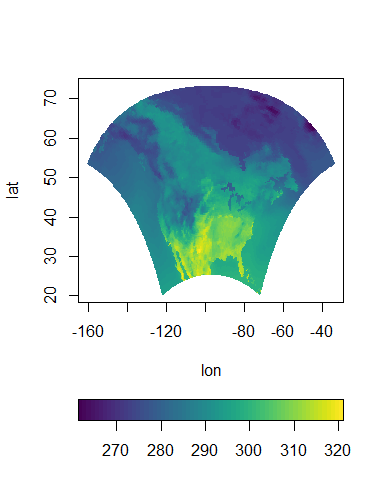x, y, and z can have differing formats depending on the type of data. If the data are observed on a regular grid, then z will be matrix with dimensions matching the dimensions of the grid and x and y will be vectors of increasing values that define the grid lines. If the data are observed on an irregular grid (e.g., a regular grid that is rotated or projected), then z will be a matrix with dimensions matching the dimensions of the grid and x and y will be matrices whose coordinates specify the x and y coordinates of each value in z. If the data are not on a grid, then x and y will be vectors specifying the coordinate locations, and z will be the vector of responses at each coordinate. If the data are not on a grid, then multilevel B-splines are used to automatically predict the response on a grid before plotting (using the mba.surf function in the MBA package).

We create a heat map using the non-gridded Aluminum measurements for the state of Colorado.

data(co, package = "gear")
pimage(co$longitude, co$latitude, co$Al, xlab = "lon", ylab = "lat")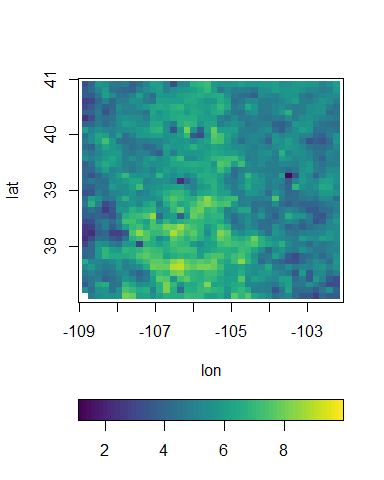We now discuss the basic options of the pimage function. The color scheme used for an image plot can be of great importance. The viridis color scheme from the viridisLite package is used by default as it is, “… designed in such a way that [it] will analytically be perfectly perceptually-uniform, both in regular form and also when converted to black-and-white. [It is] also designed to be perceived by readers with the most common form of color blindness.” The colors of the color scale can be modified by passing a vector of colors to the col argument through ..., as in the image function in graphics. We use 6 colors from the magma color palette in the viridisLite package in the next example. pimage(lon, lat, tasmax[,,1], col = viridisLite::magma(6))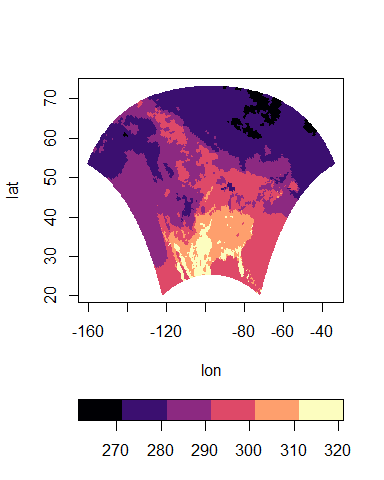The orientation of the color scale can be changed by changing the legend argument. The default is legend = "horizontal". The color scale can be removed by specifying legend = "none" or rotated to a vertical orientation by specifying legend = "vertical". The following code creates a heat map with a vertical color scale. pimage(x = lon, y = lat, z = tasmax[,,1], legend = "vertical")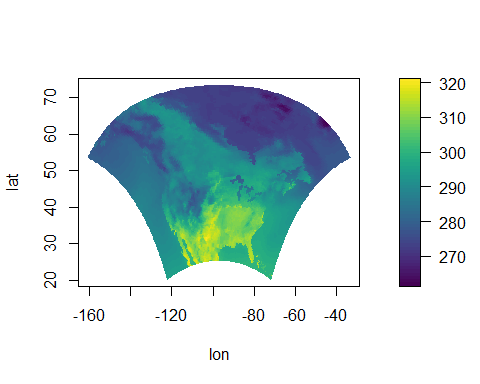The longitude and latitude coordinates can be projected before plotting by specifying the proj, parameters, and orientation arguments of pimage. Prior to plotting, the coordinates are projected using the mapproject function in the mapproj package. proj specifies the name of the projection to utilize (the default is "none"). The parameters argument specifies the parameter values of the chosen projection and orientation can be used to change the orientation of the projection. See the mapproject function in the mapproj package for more details regarding these arguments. We now create a heat map with projected coordinates. We will utilize the Bonne projection using 45 degrees as the standard parallel. A grid is automatically added to projected images because latitude and longitude parallels are not straight for most projections. pimage(x = lon, y = lat, z = tasmax[,,1], proj = "bonne", parameters = 45)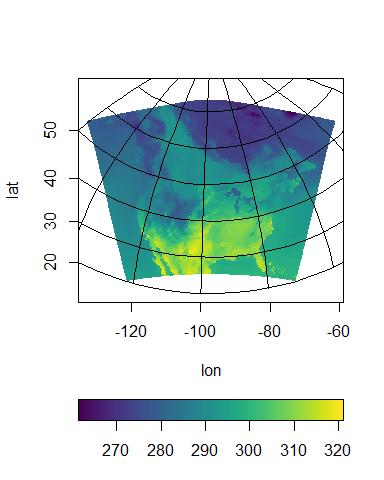Several maps can be automatically added to the image by specifying the map argument. The maps come from the maps package, and include the world, usa, state, county, france, nz (New Zealand), italy, lakes, and world2 maps. We add national boundaries to our previous map. pimage(x = lon, y = lat, z = tasmax[,,1], proj = "bonne", parameters = 45, map = "world")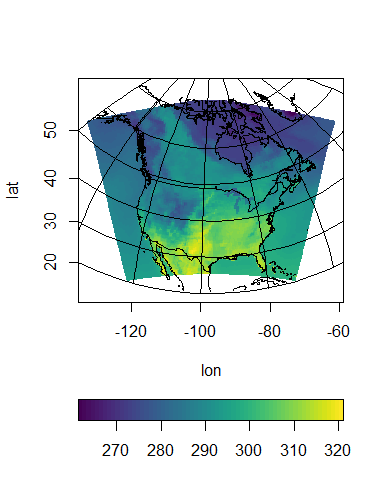The last major argument to the pimage function is the lratio argument. This argument controls the relative height or width of the color scale in comparison with the main plotting area. Increasing lratio increases the thickness of the color scale, while decreasing lratio decreases the thickness of the color scale. Additional arguments can be passed to the pimage function via .... These will be discussed at a later time in this vignette. ### Basic usage of autoimage The autoimage function generalizes the pimage function to allow for multiple images in the same plot. Most of the arguments are the same as the pimage function, and we do not replicate their discussion except when necessary. The structure of z will vary slightly from the pimage function. Specifically, if multiple gridded images are to be constructed, then z will be a three-dimensional array instead of a matrix. Each element of the third dimension of z corresponds to the matrix of gridded values for each image. If images for multiple non-gridded variables are to be constructed, then z will be a matrix with each column corresponding to a different variable. Passing a three-dimensional array to autoimage constructs a sequence of images with a common legend. autoimage(lon, lat, tasmax)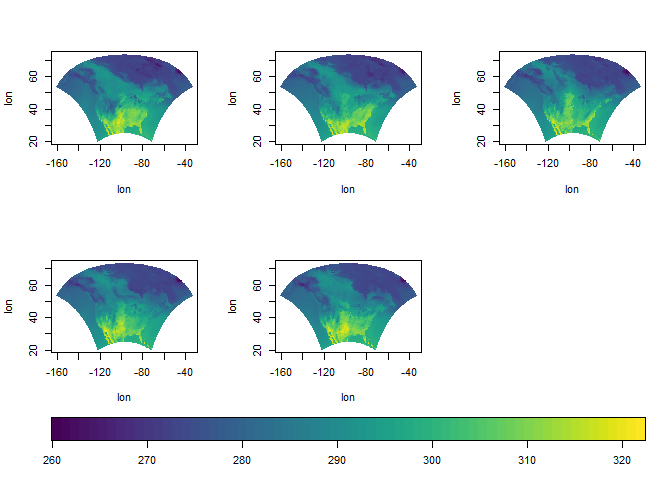Passing a two-dimensional matrix for z (where the number of rows matches the length of x and y) constructs a sequence of images for non-gridded data with a common legend. Titles are added to each image using the main argument by providing a character vector whose length matches the number of plotted images. autoimage(co$longitude, co$latitude, co[,c("Al", "Ca", "Fe", "K")], main = c("(a) Aluminum %", "(b) Calcium %", "(c) Iron %", "(d) Potassium %"), xlab = "lon", ylab = "lat")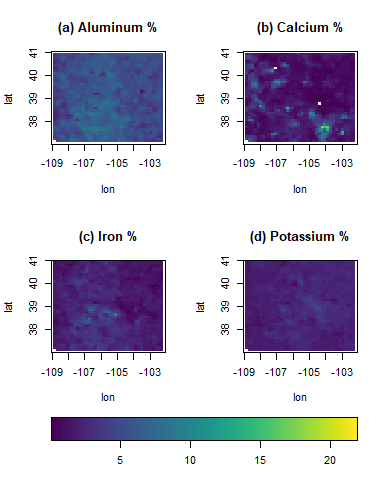Separate color scales will be used for each image when common.legend = FALSE. autoimage(co$longitude, co$latitude, co[,c("Al", "Ca", "Fe", "K")], common.legend = FALSE, main = c("(a) Aluminum %", "(b) Calcium %", "(c) Iron %", "(d) Potassium %"), xlab = "lon", ylab = "lat")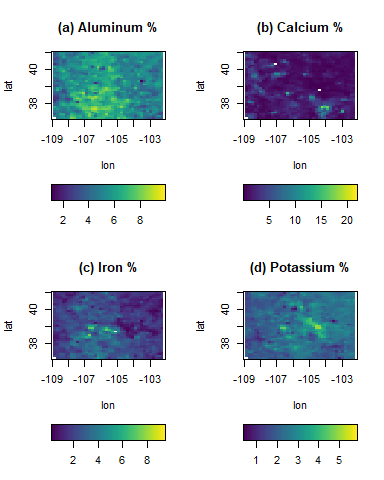The dimensions of the plotting matrix can be changed by specifying the size argument. If not provided, then a sensible choice is automatically chosen via the autosize function. The size argument should be a two-dimensional vector where the first element corresponds to the number of rows of images and the second dimension corresponds to the number of columns. We create a 1 $$\times$$ 3 matrix of images for the NARCCAP data. autoimage(lon, lat, tasmax[,,1:3], size = c(1, 3))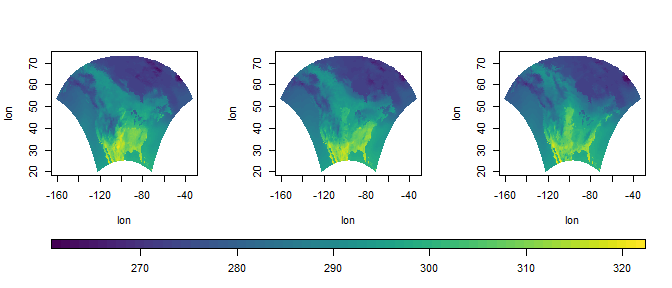A common title is sometimes desired for a sequence of images. This can easily be added by specifying the outer.title argument. The margins of the common title can be controlled via the oma argument of par. However, if oma is not specified beforehand, then a sensible value is automatically chosen (while showing a warning to the user.) We add a common title to the NARCCAP data. autoimage(lon, lat, tasmax, outer.title = "tasmax for 5 days") ## Warning in autolayout(size, legend = legend, common.legend = common.legend, : There is no room in the outer margin for an outer title. ## Setting par(oma = c(0, 0, 3, 0)).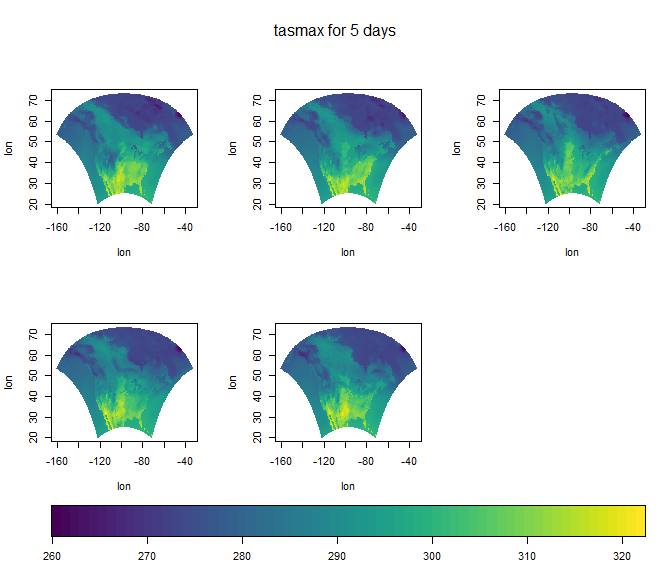The mercator projection can sometimes be problematic for various reasons, especially with the world map as horizontal lines appear across the plot area. We have attempted to solve this issue by clipping x and y coordinates that are outside the range of xlim and ylim. autoimage(x = lon, y = lat, z = tasmax[,,1], map = "world", xlab = "longitude", ylab = "latitude", proj = "mercator", axes = FALSE)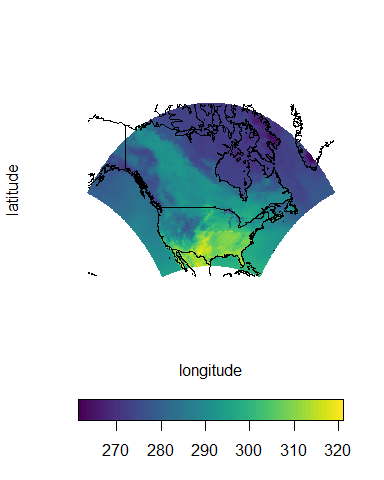### Richer plots using autolayout and autolegend Suppose we want to add custom features to a sequences of images, with each image receiving different features. One can create a richer sequence of images using the autolayout and autolegend functions. The autolayout function partitions the graphic device into the sections needed to create a sequence of images. The most important function arguments include size, legend, common.legend, and lratio, which correspond to the same arguments in the autoimage function. The outer argument specifies whether an outer.title is desired. The default is FALSE. By default, numbers identify the plotting order of the sections, though these can be hidden by setting show = FALSE. As an initial example, we create a 2 $$\times$$ 3 grid of images with a common vertical legend. autolayout(c(2, 3), legend = "v")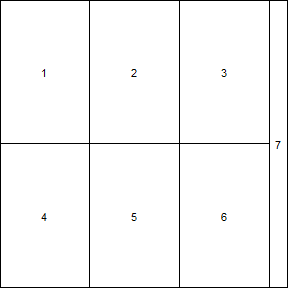The images should be created using the pimage function while specifying legend = "none". After the desired image or set of images is created, one can automatically add the appropriate legend by calling autolegend. The autolegend function recovers relevant legend parameters from the most recent pimage call. Consequently, if a common legend is desired, then it is important to specify a common zlim argument among all relevant pimage calls. Various features can be added to the images using the ppoints, plines, ptext, psegments, parrows, and ppolygon functions. These are analogues of the points, lines, text, segments, arrows, and polygon functions in the graphics package, to be used with images containing projected coordinates. We now create a complicated (though unrealistic) example of this. We first extract the borders of Hawaii and Alaska from the "world" map in the maps package and store it as the hiak list. We then select a small subset of cities in Colorado from the us.cities dataset in the maps package and store this in the codf data frame. Lastly, we select the U.S. capitals from the us.cities dataset and store this in the capdf data frame. # load world map data(worldMapEnv, package = "maps") # extract hawaii and alaskan borders hiak <- maps::map("world", c("USA:Hawaii", "USA:Alaska"), plot = FALSE) # load us city information data(us.cities, package = "maps") # extract colorado cities from us.cities codf <- us.cities[us.cities$country.etc == "CO", ]
# select smaller subset of colorado cities
# extract capitals from us.cities
capdf <- us.cities[us.cities$capital == 2,] Having obtained the relevant information, we setup a 1 $$\times$$ 2 matrix of images with individual horizontal legends and an area for a common title. We create an image plot of NARCCAP data using the mercator projection and including grey state borders. The borders of Hawaii and Alaska are added using the plines function. The state capitals are added to the image using the ppoints function. The first image is then titled using the title function. The legend is then added using the autolegend function. Next, an image of the Colorado Aluminum measurements is created. The coordinates are projected using the Bonne projection, the color scheme is customized, grey county borders are added to the plot, but the grid lines are removed. The ppoints function is then used to add locations for several Colorado cities to the image. The ptext function is then used to add the names of these cities to the image. The second image is then titled using the title function. The appropriate legend is then added using the autolegend function. Lastly, a common title is added using the mtext function. # setup plotting area autolayout(c(1, 2), legend = "h", common.legend = FALSE, outer = TRUE) ## Warning in autolayout(c(1, 2), legend = "h", common.legend = FALSE, outer = TRUE): There is no room in the outer margin for an outer title. ## Setting par(oma = c(0, 0, 3, 0)).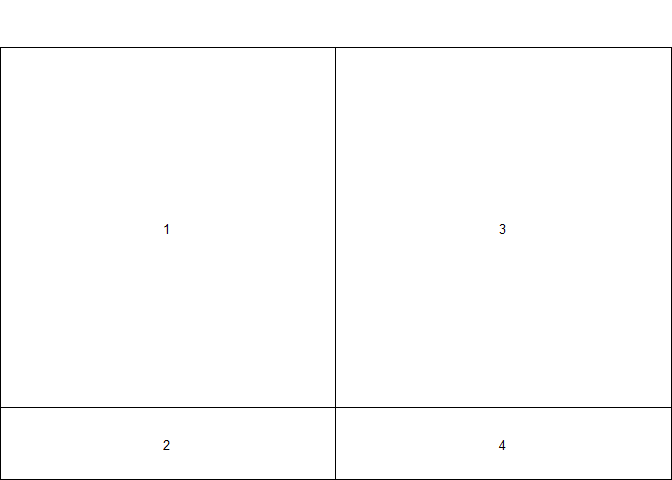# create image of NARCCAP data. # xlim is chosen so to include alaska and hawaii # add grey state borders pimage(lon, lat, tasmax[,,1], legend = "none", proj = "mercator", map = "state", xlim = c(-180, 20), lines.args = list(col = "grey")) ## Warning in paxes(xlim = c(-180, 20), ylim = c(20.5263919830322, ## 73.0147552490234: The x axis tick positions are not between -180 and 180, which ## creates problems with the mercator projection. Attempting to automatically ## correct the issue. The user may need to specify xaxp, or for more control, the ## xat argument of the paxes.args list. # add hawaii and alaskan borders plines(hiak, proj = "mercator", col = "grey") # add state captials to image ppoints(capdf$lon, capdf$lat, proj = "mercator", pch = 16) # title image title("tasmax for North America") # add legend for plot autolegend() # load colorado geochemical data data(co, package = "gear") # create image for colorado aluminum measurements # use bonne projection # customize legend colors # add grey county borders # exclude grid pimage(co$lon, co$lat, co$Al, map = "county", legend = "none",
proj = "bonne", parameters = 39,
paxes.args = list(grid = FALSE),
col = fields::tim.colors(64),
lines.args = list(col = "grey"),
xlab = "lon", ylab = "lat")
ppoints(codf$lon, codf$lat, pch = 16, proj = "bonne")
ptext(codf$lon, codf$lat, labels = codf$name, proj = "bonne", pos = 4) # title plot title("Colorado Aluminum levels (%)") # add legend to current image autolegend() # add common title for plots mtext("Two complicated maps", col = "purple", outer = TRUE, cex = 2)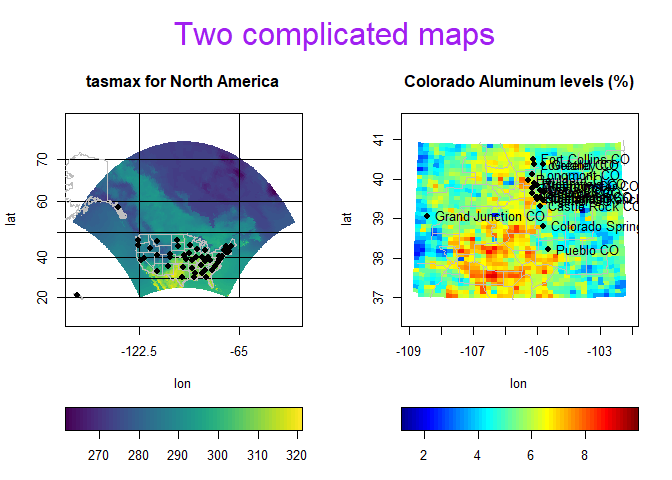### More advanced options in the pimage and autoimage functions The plots generated by the pimage and autoimage functions can be customized in numerous ways by passing additional arguments through the ... argument of the functions. The customizations are mostly the same for both functions, so we illustrate these customizations using the pimage function when possible for simplicity. Lines or points can be added to each image by passing the lines and points arguments to the functions. Each argument should be a named list with x and y components specifying the coordinates to join (for lines) or plot for points. Note that if multiple unconnected lines are to be drawn, then each line should be separated by an NA value, similar to how maps are constructed in the maps package. To illustrate usage of these arguments, we extract United States state boundaries from the maps package and reformat the us.cities dataset from the maps package. Note that statepoly is automatically a list with x and y components, while this must be created manually for the us.cities dataset. data(stateMapEnv, package = "maps") statepoly <- maps::map("state", plot = FALSE) citylist <- list(x = us.cities$long, y = us.cities$lat) We now add these state lines and city locations to the image. pimage(lon, lat, tasmax[,,1], lines = statepoly, points = citylist)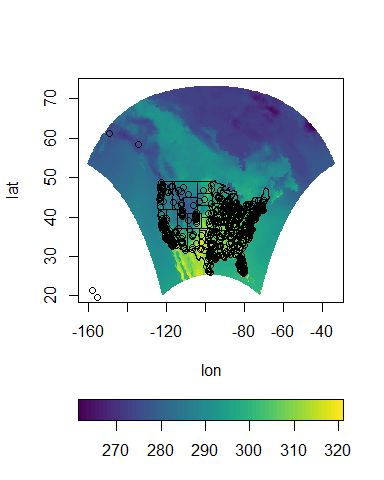The appearance of the lines and points can be customized by passing the lines.args and points.args arguments through .... Each argument should be a named list with components matching the arguments of the lines and points functions in the graphics package. We change the appearance of the lines and points in the previous plot by specifying these arguments. pimage(lon, lat, tasmax[,,1], lines = statepoly, points = citylist, lines.args = list(lwd = 2, lty = 3, col = "white"), points.args = list(pch = 20, col = "blue"))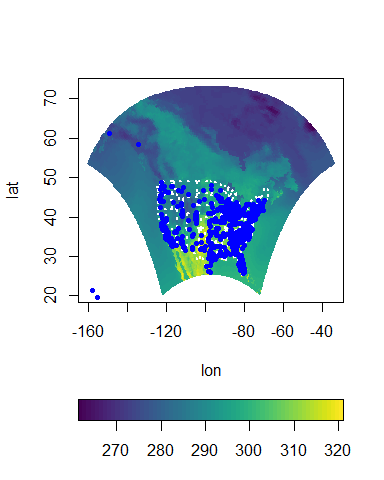Text can be added to each image by passing the text argument through .... text should be a named list with components x and y, which specify the locations to draw the text, and labels, which specifies the text to write at each location. The appearance of the text can be customized by passing the text.args argument. text.args should be a named list with components matching the non-x, y, and labels arguments of the text function in the graphics package. We add the names and locations of two Colorado cities to the Colorado geochemical data. citypoints = list(x = c(-104.98, -104.80), y = c(39.74, 38.85), labels = c("Denver", "Colorado Springs")) autoimage(co$lon, co$lat, co[,c("Al", "Ca")], common.legend = FALSE, main = c("Aluminum", "Cadmium"), points = citypoints, points.args = list(pch = 20, col = "white"), text = citypoints, text.args = list(pos = 3, col = "white"), xlab = "lon", ylab = "lat")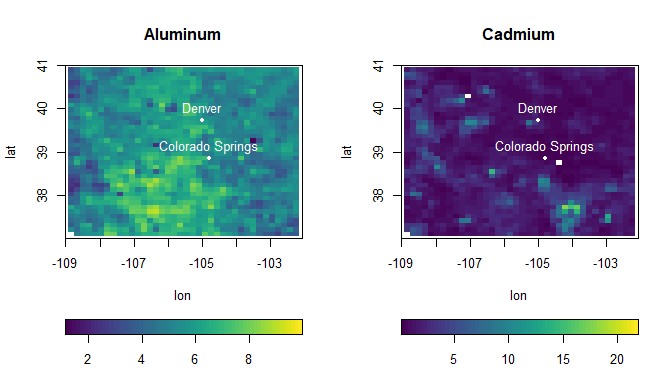When projections are used, the grid lines do not always go as far as they should. Thus, axis customization is desired. Additionally, the appearance of the grid lines might need improving. The appears of the axis (and the locations of the grid lines) can be changed by passing axis.args through .... axis.args should be a named list with components matching the arguments of the axis function in graphics. The exception is that xat and yat arguments are used instead of at so that ticks on the x and y axes can be specified separately. The appearance of the grid lines can be changed by passing the desired changes through the paxes.args argument. This is a named list with components matching the arguments in the lines function. Consider the following poor graphic. pimage(lon, lat, tasmax[,,1], proj = "bonne", parameters = 40)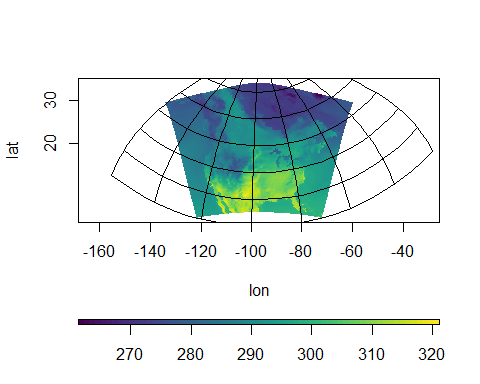The grid lines do not extend nearly far enough. There are only two tick marks on the y axis. The legend is too thin. We can add additional longer grid lines by specifying xat and yat in axis.args. We can also further change the appearance of the axes via other components of axis.args. We change the appearance of the grid lines by specifying choices in paxes.args. We change the appearance of the legend by specifying choices in legend.axis.args. We change the legend thickness by specifying lratio. pimage(lon, lat, tasmax[,,1], proj = "bonne", parameters = 40, axis.args = list(yat = seq(-10, 70, by = 10), xat = seq(-220, 20, by = 20), col.axis = "darkgrey", cex.axis = 0.9), paxes.args = list(col = "grey", lty = 2), legend.axis.args = list(cex.axis = 0.9), lratio = 0.3)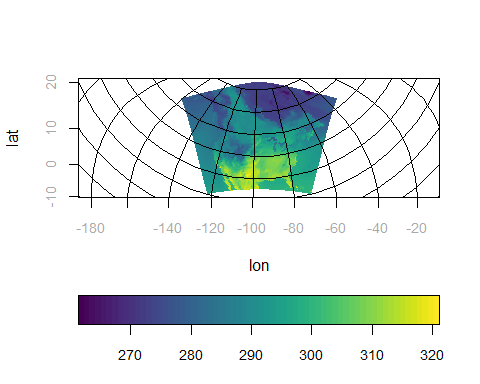The breaks and colors of the scale can be modified by specifying the breaks and col arguments, as in the image function in graphics. Additional changes can be made by specifying legend.axis.args, a named list with components matching the arguments of the axis function, which is used in creating the legend. pimage(lon, lat, tasmax[,,1], col = viridisLite::magma(6), breaks = c(0, 275, 285, 295, 305, 315, 325), legend.axis.args = list(col.axis = "blue", las = 2, cex.axis = 0.75))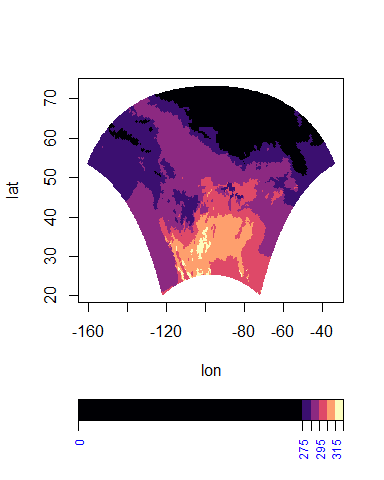When non-gridded data are used, the settings for the gridded surface approximation can be passed through ... by specifying interp.args, a named list with components matching the non-xyz arguments of the mba.surf function in the MBA package. We project the Colorado Aluminum measurements onto a finer grid in the following plot. pimage(co$lon, co$lat, co$Al, interp.args = list(no.X = 100, no.Y = 100),
xlab = "lon", ylab = "lat")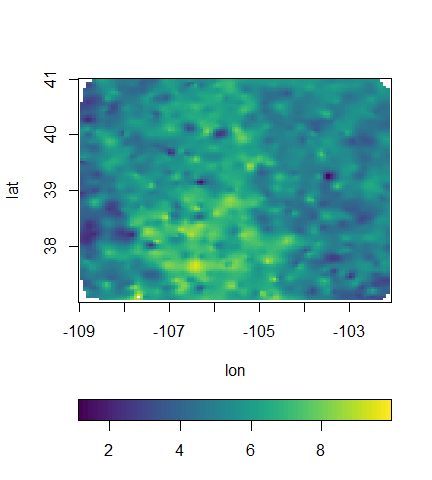The appearance of outer.title can be modified by passing mtext.args through .... mtext.args should be a named list with components matching the arguments of mtext, which is the function used to create the common title.

autoimage(lon, lat, tasmax, outer.title = "tasmax for 5 days",
mtext.args = list(col = "blue", cex = 2))
## Warning in autolayout(size, legend = legend, common.legend = common.legend, : There is no room in the outer margin for an outer title.
##               Setting par(oma = c(0, 0, 3, 0)).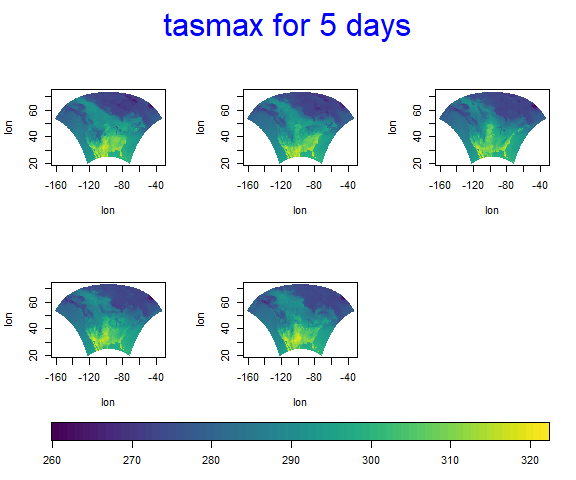The various options of the labeling, axes, and legend are largely independent. e.g., passing col.axis through ... will not affect the axis unless it is passed as part of the named list axis.args. However, one can set the various par options prior to plotting to simultaneously affect the appearance of multiple aspects of the plot.
After plotting, reset.par can be used to reset the graphics device options to their default values. We provide an example below.

par(cex.axis = 0.5, cex.lab = 0.5, mgp = c(1.5, 0.5, 0),
mar = c(2.1, 2.1, 2.1, 0.2), col.axis = "orange",
col.main = "blue", family = "mono")
pimage(lon, lat, tasmax[,,1])
title("very customized plot")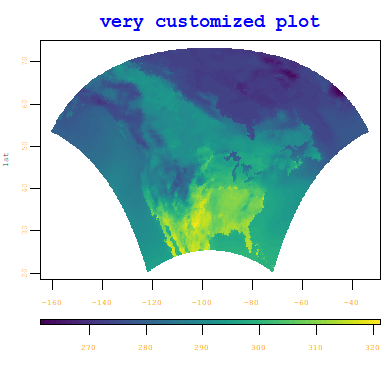reset.par()